# Java reference mechanism (stack and heap)

I had the opportunity to explain the Java reference mechanism to my juniors using the stack area and Java heap area. I remember being confused about Java references when I didn't know the relationship between these areas. It's a good opportunity, so I'll summarize it in an article.

## Mechanism to realize Java reference

The JVM has a stack area and a Java heap area to realize Java reference. Java references are realized by these two areas.

Area name Overview
Stack area It mainly holds reference information to the heap area. It also holds the value of the primitive type.
JAVA heap area The actual value of the object is kept in this memory.

## Differences in how objects and primitive type values are retained

Java objects (Class, array, etc.) have heap area reference information in the stack area. The actual value is stored in the heap area.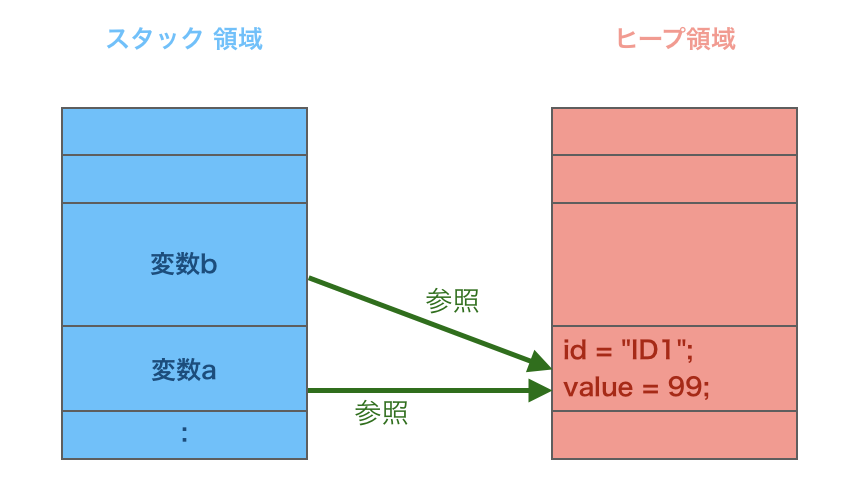Primitive types have different memory management than objects. When you create a variable of primitive type, the value is held in the stack area.

• However, since the primitive type array is treated as an object, the value is retained in the heap area.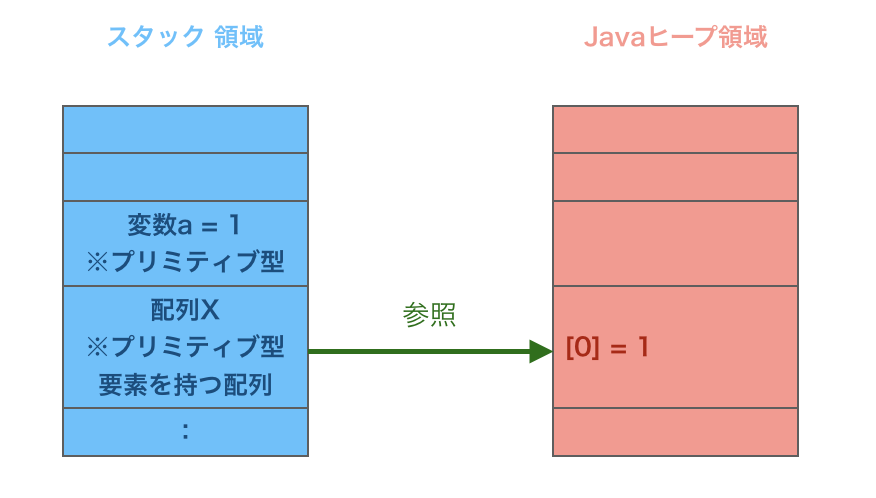## Behavior when passing to method arguments

When passing an object or primitive type variable to a method argument The contents of the stack area are copied to another stack area.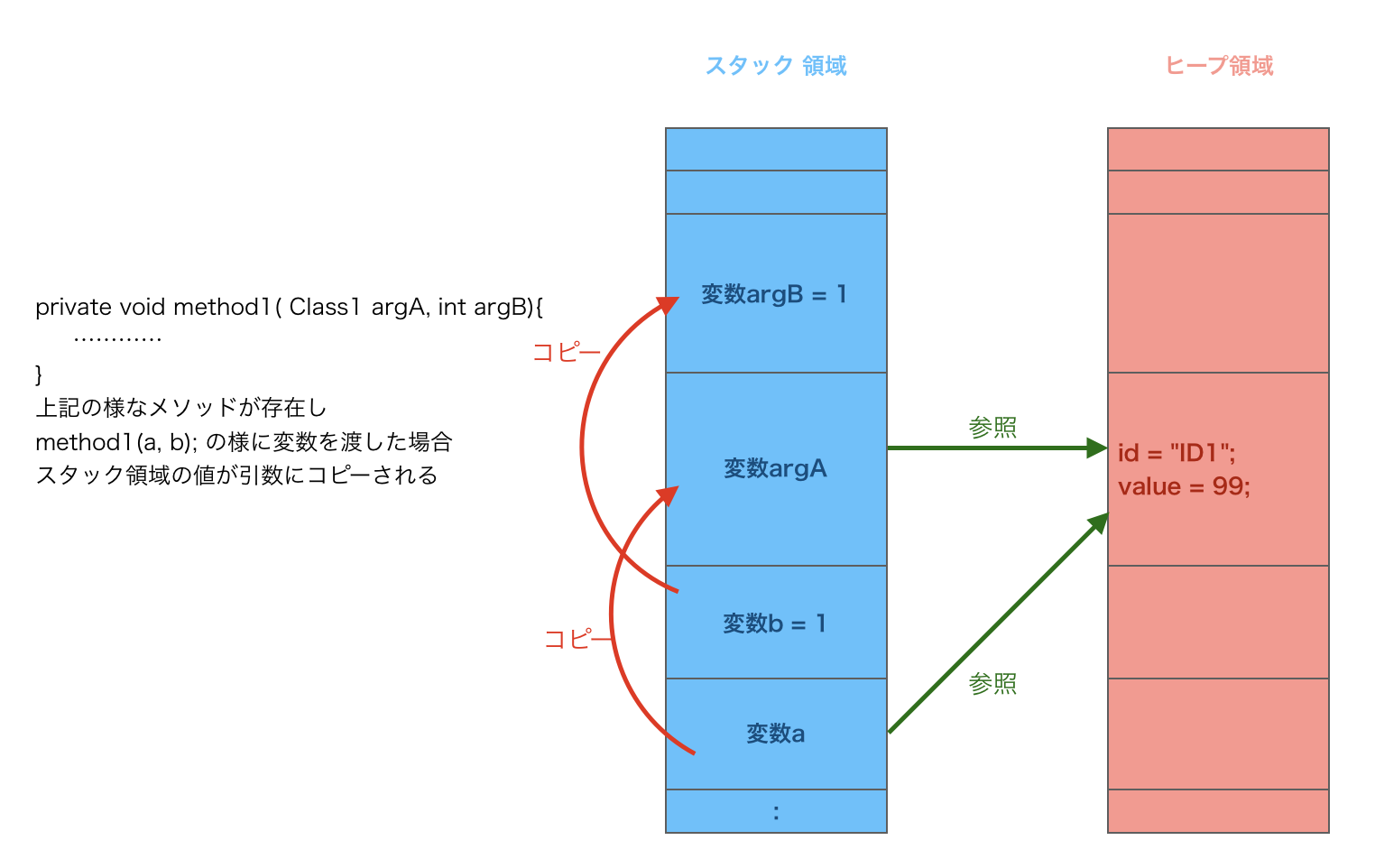In the above case, the reference of argA is the same as the variable a. Changing the referenced value inside method1 also affects the variable a.

• Since argB is a primitive type, it is separated from the variable b.

## Source code sample

In order to confirm that the contents described so far are correct, we will explain by comparing the source code with the output result.

### Example 1

#### `Source code`

``````
package test;

class Class1 {
String id;
int value;
}

public class Test {
public static void main(String[] args) {

Class1 a = new Class1();
a.id = "ID1";
a.value = 1;

//Operation 
Class1 b = a;
//Operation 
b.id = "ID2";
b.value = 2;

//Operation 
b = null;
//What is the value output here?
System.out.println("id:" + a.id);
System.out.println("value:" + a.value);
}
}
``````

[Operation details]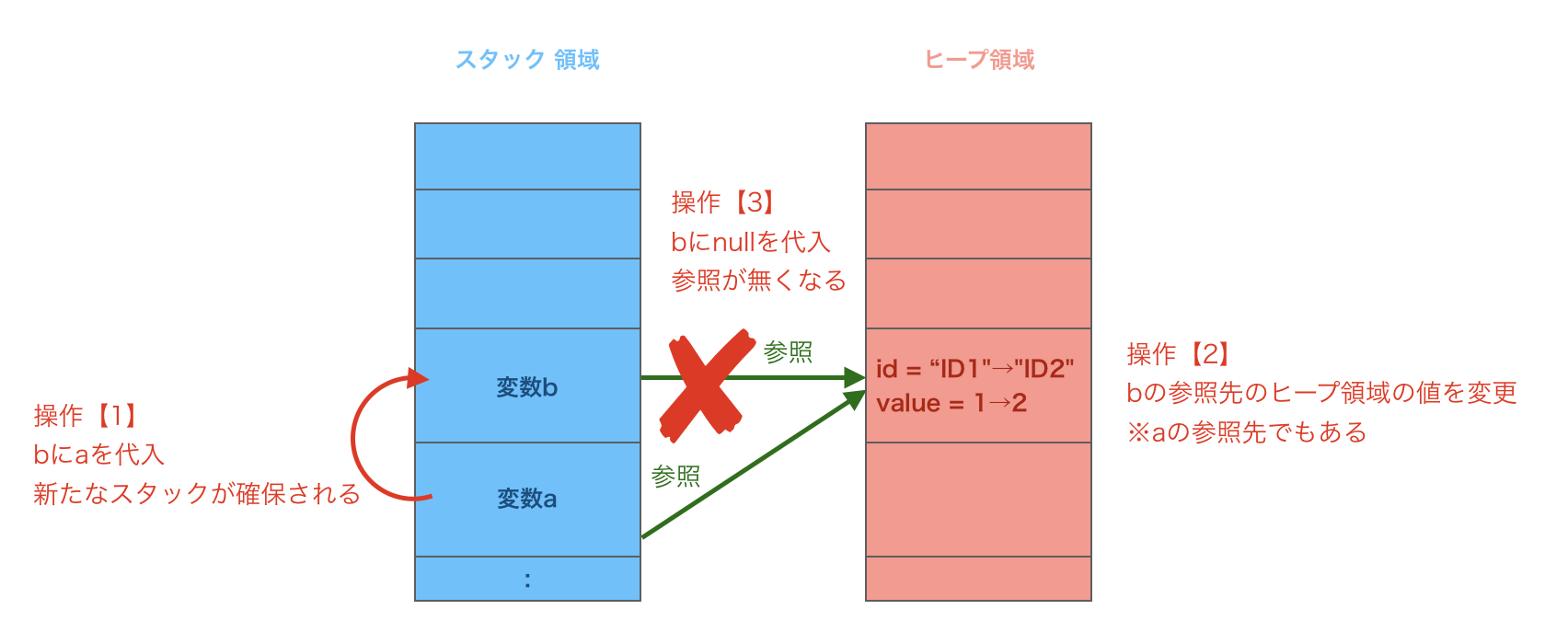By operation , the value of the reference destination of variable a is also rewritten. Operation  deletes the reference to variable b, but it does not affect variable a with a different stack. Therefore, the output contents are as follows.

#### `Output result`

``````
id:ID2
value:2
``````

### Example 2

#### `Source code`

``````
package test;

class Class1 {
String id;
int value;
}

public class Test {
public static void main(String[] args) {

Class1 a = new Class1();
a.id = "ID1";
a.value = 1;

//Operation 
method1(a);
//What is the value output here?
System.out.println("id:" + a.id);
System.out.println("value:" + a.value);
}

private static void method1(Class1 argA) {
//Operation 
argA.id = "ID2";
argA.value = 2;
}
}
``````

[Operation details]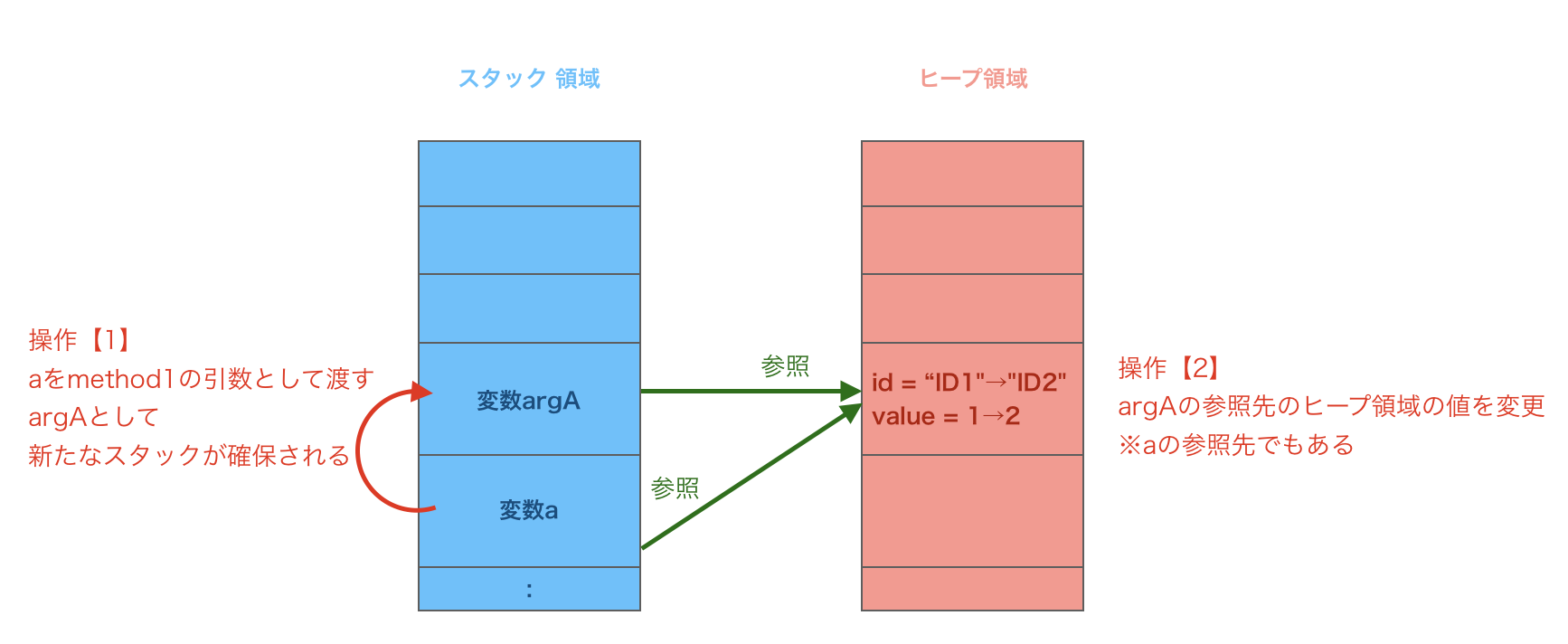By operation , the value of the reference destination of variable a is also rewritten. Therefore, the output contents are as follows.

#### `Output result`

``````
id:ID2
value:2
``````

### Example 3

#### `Source code`

``````
package test;

class Class1 {
String id;
int value;
}

public class Test {
public static void main(String[] args) {

Class1 a = new Class1();
a.id = "ID1";
a.value = 1;

//Operation 
method1(a);
//What is the value output here?
System.out.println("1st time =========");
System.out.println("id:" + a.id);
System.out.println("value:" + a.value);

//Operation 
method2(a);
//What is the value output here?
System.out.println("Second time =========");
System.out.println("id:" + a.id);
System.out.println("value:" + a.value);
}

private static void method1(Class1 argA) {
argA = null;
}

private static void method2(Class1 argA) {
argA = new Class1();
argA.id = "ID2";
argA.value = 2;
}
}
``````

[Operation details] As in Example 2, we are passing the variable a as a method argument. The method of operation  assigns null and deletes the reference of argA, but it does not affect the variable a with a different stack. Since the method of operation  is new to Class1, the reference destination has changed to the variable a. Setting the id and value values after the reference has changed does not affect the variable a. Therefore, the output contents are as follows.

#### `Output result`

``````
1st time =========
id:ID1
value:1
Second time =========
id:ID1
value:1
``````

### Example 4

#### `Source code`

``````
package test;

public class Test {
public static void main(String[] args) {

int a = 1;

//Operation 
int b = a;
//Operation 
b = 99;

//What is the value output here?
System.out.println("1st time =========");
System.out.println("a:" + a);
System.out.println("b:" + b);

//Operation 
method1(a);

//What is the value output here?
System.out.println("Second time =========");
System.out.println("a:" + a);
}

private static void method1(int argA) {
//Operation 
argA = 99;
}
}
``````

[Operation details]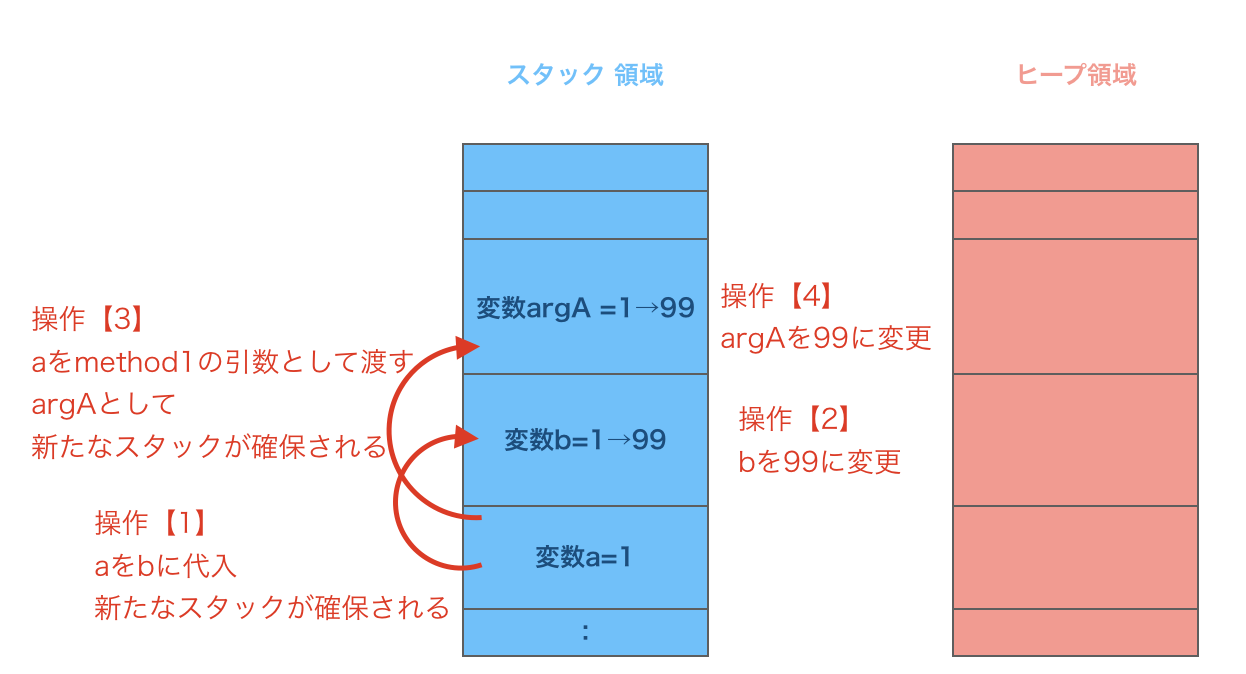Each primitive type variable holds its value in the stack area independently. Each operation does not affect another variable. Therefore, the output contents are as follows.

#### `Output result`

``````
1st time =========
a:1
b:99
Second time =========
a:1
``````

### Example 5

#### `Source code`

``````
package test;

public class Test {
public static void main(String[] args) {

int a = 0;

//Operation 
int[] b = {a};

//Operation 
method1(b);

//What is the value output here?
System.out.println("a:" + a);
System.out.println("b:" + b);
}

private static void method1(int[] argB) {
//Operation 
argB = 99;
}
}
``````

[Operation details]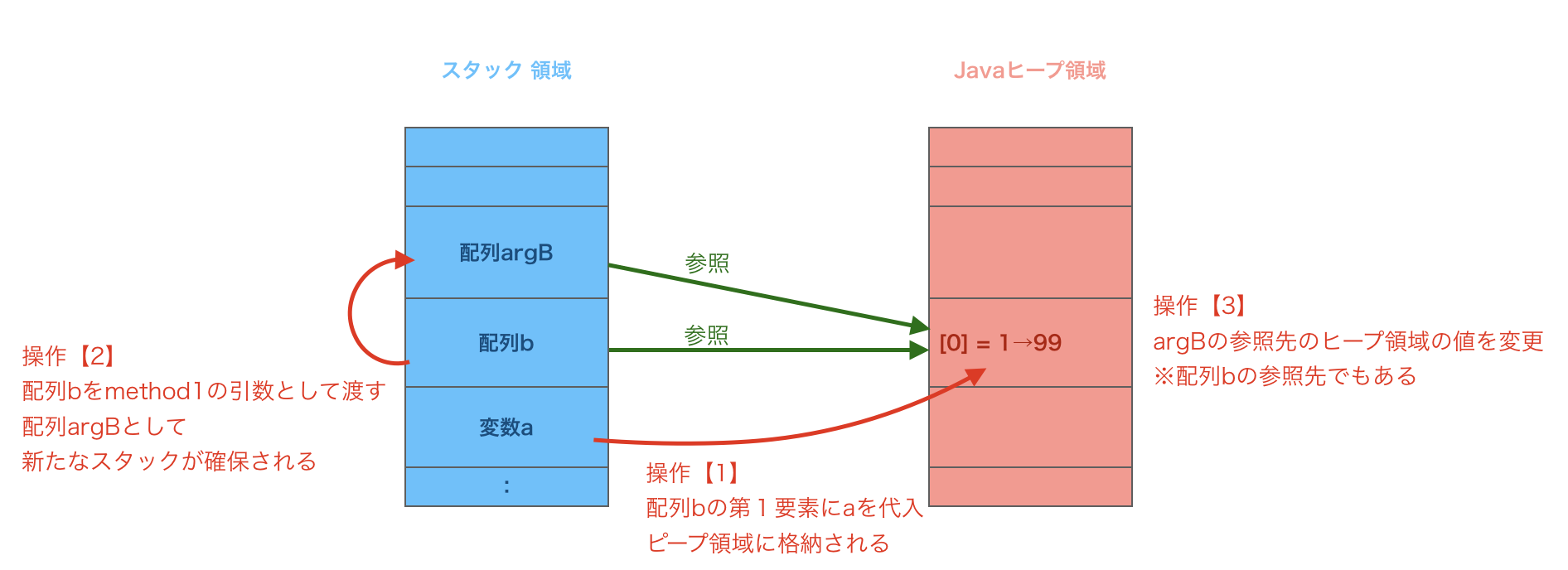Even if it is a primitive type, if you keep it as an array, its value will be saved in the heap area. If you change the array argB in operation , it will affect the array b that has the same reference destination. Therefore, the output contents are as follows.

#### `Output result`

``````
a:0
b:99
``````